# TensorFlow学习笔记之快速求解四元一次方程的完整代码以及保存模型和读取模型的讲解

### 二、训练代码

# 训练代码
import tensorflow as tf
import numpy as np
import time

start = time.clock()

# 生成数据
np.random.seed(0)
outputs = np.random.randint(5, size=(10, 4))
res = []
for i in range(10):
# 假设方程式为 y=4a+6b+7c+2d
y = 4 * outputs[i] + 6 * outputs[i] + \
7 * outputs[i] + 2 * outputs[i]
res.append([y])

# 定义数据
train_data = np.array(outputs).astype('float32')
y_true = np.array(res).astype('float32')

# 占位符
x = tf.placeholder(tf.float32, shape=[None, 4], name='x')
y = tf.placeholder(tf.float32, shape=[None, 1], name='y')

# w 是要求的各个参数的权重，是目标输出，对应 t_w
w = tf.Variable(np.ones(4, dtype=np.float32).reshape((4, 1)), tf.float32)

# 实际输出数据
y_ = tf.matmul(x, w)

# 定义损失函数，均方误差
loss = tf.reduce_mean(tf.square(y - y_))

# 定义一个梯度下降算法来进行训练的优化器

# 最小化代价函数
train = optimizer.minimize(loss)

# 初始化变量
init = tf.global_variables_initializer()

# 开始训练
with tf.Session() as sess:
sess.run(init)
for step in range(500):
_, curr_loss = sess.run([train,loss], feed_dict={x: train_data, y: y_true})
if step % 50 == 0:
print("运行%d 次,loss=%s" % (step,curr_loss))
#用saver 保存模型
saver = tf.train.Saver()
saver.save(sess, "model_data/model")
elapsed = (time.clock() - start)
print("Training Time used:",elapsed)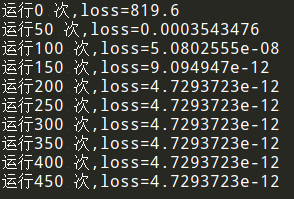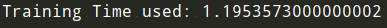#### 1、代码讲解：计算运行时间

import time

start = time.clock()
......
......
elapsed = (time.clock() - start)
print("Training Time used:",elapsed)


#### 2、代码讲解：占位符

# 占位符
x = tf.placeholder(tf.float32, shape=[None, 4], name='x')
y = tf.placeholder(tf.float32, shape=[None, 1], name='y')


tf.placeholder(dtype, shape=None, name=None)


• dtype：数据类型。常用的是tf.float32,tf.float64等数值类型
• shape：数据形状。默认是None，就是一维值，也可以是多维（比如[2,3], [None, 3]表示列是3，行不定）
• name：名称

#### 3、代码讲解：网络结构搭建

# w 是要求的各个参数的权重，是目标输出，对应 t_w
w = tf.Variable(np.ones(4, dtype=np.float32).reshape((4, 1)), tf.float32)

# 实际输出数据
y_ = tf.matmul(x, w)


#### 4、代码讲解：损失函数和优化器

# 定义损失函数，均方误差
loss = tf.reduce_mean(tf.square(y - y_))

# 定义一个梯度下降算法来进行训练的优化器

# 最小化代价函数
train = optimizer.minimize(loss)


#### 5、代码讲解：开始训练

# 初始化变量
init = tf.global_variables_initializer()

# 开始训练
with tf.Session() as sess:
sess.run(init)
for step in range(500):
_, curr_loss = sess.run([train,loss], feed_dict={x: train_data, y: y_true})
if step % 50 == 0:
print("运行%d 次,loss=%s" % (step,curr_loss))


#### 6、代码讲解：保存模型

#用saver 保存模型
saver = tf.train.Saver()
saver.save(sess, "model_data/model")


tensorflow 提供了 tf.train.Saver 类来保存模型，值得注意的是，在tensorflow中，变量是存在于Session环境中，也就是说，只有在Session环境下才会存有变量值，因此，保存模型时需要传入session。

tf.train.Saver(max_to_keep=5, keep_checkpoint_every_n_hours=2)


### 三、测试代码

# 测试代码
import tensorflow as tf
import numpy as np

def main():
sess = tf.InteractiveSession()
# 加载模型
saver = tf.train.import_meta_graph('model_data/model.meta')
saver.restore(sess, 'model_data/model')
graph = tf.get_default_graph()

# 得到当前图中所有变量的名称
# tensor_name_list = [tensor.name for tensor in graph.as_graph_def().node]
# print(tensor_name_list)

x = graph.get_tensor_by_name('x:0')
pre_result = graph.get_tensor_by_name("MatMul:0")

# 生成测试数据
test = np.array([[9,5,2,10]]).astype('float32')

# 进行预测
pre_reload_out = sess.run(pre_result, feed_dict={x: test})

# 关闭会话
sess.close()

if __name__ == '__main__':
main()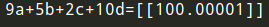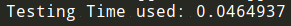#### 1、代码讲解：读取模型

sess = tf.InteractiveSession()
# 加载模型
saver = tf.train.import_meta_graph('model_data/model.meta')
saver.restore(sess, 'model_data/model')
graph = tf.get_default_graph()


|--checkpoint_dir
|    |--checkpoint
|    |--MyModel.meta
|    |--MyModel.data-00000-of-00001
|    |--MyModel.index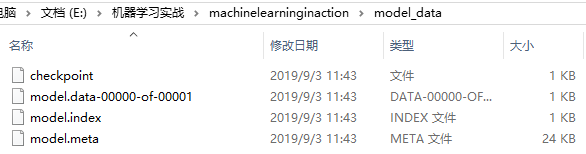1. meta文件

model.meta文件保存的是图结构，meta文件是pb（protocol buffer）格式文件，包含变量、op、集合等。

1. ckpt文件

ckpt文件是二进制文件，保存了所有的weights、biases、gradients等变量。在tensorflow 0.11之前，保存在 .ckpt 文件中。0.11后，通过两个文件保存,如：

model.data-00000-of-00001
model.index

1. checkpoint文件

checkpoint_dir目录下还有checkpoint文件，该文件是个文本文件，里面记录了保存的最新的checkpoint文件以及其它checkpoint文件列表。在inference时，可以通过修改这个文件，指定使用哪个model

#### 2、代码讲解：变量名获取

#得到当前图中所有变量的名称
tensor_name_list = [tensor.name for tensor in graph.as_graph_def().node]
print(tensor_name_list)


['x', 'y', 'Variable/initial_value', 'Variable', 'Variable/Assign', 'Variable/read', 'MatMul', 'sub',
'Square', 'Const', 'Mean', 'gradients/Shape'......]


#### 3、代码讲解：加载参数

x = graph.get_tensor_by_name('x:0')
pre_result = graph.get_tensor_by_name("MatMul:0")


#### 4、代码讲解：预测结果

# 进行预测
pre_reload_out = sess.run(pre_result, feed_dict={x: test})


### 总结